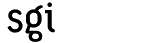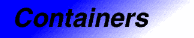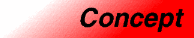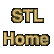# SequenceCategory: containers Component type: concept

### Description

A Sequence is a variable-sized Container whose elements are arranged in a strict linear order. It supports insertion and removal of elements.

### Refinement of

Forward Container, Default Constructible

### Associated types

None, except for those of Forward Container.

### Notation

 X A type that is a model of Sequence a, b Object of type X T The value type of X t Object of type T p, q Object of type X::iterator n Object of a type convertible to X::size_type

### Definitions

If a is a Sequence, then p is a valid iterator in a if it is a valid (nonsingular) iterator that is reachable from a.begin().

If a is a Sequence, then [p, q) is a valid range in a if p and q are valid iterators in a and if q is reachable from p.

### Valid expressions

In addition to the expressions defined in Forward Container, the following expressions must be valid.
Name Expression Type requirements Return type
Fill constructor X(n, t)   X
Fill constructor X a(n, t);
Default fill constructor X(n) T is DefaultConstructible. X
Default fill constructor X a(n); T is DefaultConstructible.
Range constructor X(i, j) i and j are Input Iterators whose value type is convertible to T  X
Range constructor X a(i, j); i and j are Input Iterators whose value type is convertible to T 
Front a.front()   reference if a is mutable, const_reference otherwise.
Insert a.insert(p, t)   X::iterator
Fill insert a.insert(p, n, t) a is mutable void
Range insert a.insert(p, i, j) i and j are Input Iterators whose value type is convertible to T . a is mutable void
Erase a.erase(p) a is mutable iterator
Range erase a.erase(p,q) a is mutable iterator
Clear a.clear() a is mutable void
Resize a.resize(n, t) a is mutable void
Resize a.resize(n) a is mutable void

### Expression semantics

Semantics of an expression is defined only where it is not defined in Forward Container, or where it differs.
Name Expression Precondition Semantics Postcondition
Fill constructor X(n, t) n >= 0 Creates a sequence with n copies of t size() == n. Every element is a copy of t.
Fill constructor X a(n, t); n >= 0 Creates a sequence with n copies of t a.size() == n. Every element of a is a copy of t.
Default fill constructor X(n) n >= 0 Creates a sequence of n elements initialized to a default value. size() == n. Every element is a copy of T().
Default fill constructor X a(n, t); n >= 0 Creates a sequence with n elements initialized to a default value. a.size() == n. Every element of a is a copy of T().
Default constructor X a; or X()   Equivalent to X(0). size() == 0.
Range constructor X(i, j) [i,j) is a valid range. Creates a sequence that is a copy of the range [i,j) size() is equal to the distance from i to j. Each element is a copy of the corresponding element in the range [i,j).
Range constructor X a(i, j); [i,j) is a valid range. Creates a sequence that is a copy of the range [i,j) a.size() is equal to the distance from i to j. Each element in a is a copy of the corresponding element in the range [i,j).
Front a.front() !a.empty() Equivalent to *(a.first())
Insert a.insert(p, t) p is a valid iterator in a. a.size() < a.max_size() A copy of t is inserted before p.   a.size() is incremented by 1. *(a.insert(p,t)) is a copy of t. The relative order of elements already in the sequence is unchanged.
Fill insert a.insert(p, n, t) p is a valid iterator in a. n >= 0 && a.size() + n <= a.max_size(). n copies of t are inserted before p.    a.size() is incremented by n. The relative order of elements already in the sequence is unchanged.
Range insert a.insert(p, i, j) [i,j) is a valid range. a.size() plus the distance from i to j does not exceed a.max_size(). Inserts a copy of the range [i,j) before p.    a.size() is incremented by the distance from i to j. The relative order of elements already in the sequence is unchanged.
Erase a.erase(p) p is a dereferenceable iterator in a. Destroys the element pointed to by p and removes it from a.  a.size() is decremented by 1. The relative order of the other elements in the sequence is unchanged. The return value is an iterator to the element immediately following the one that was erased.
Range erase a.erase(p,q) [p,q) is a valid range in a. Destroys the elements in the range [p,q) and removes them from a.  a.size() is decremented by the distance from i to j. The relative order of the other elements in the sequence is unchanged. The return value is an iterator to the element immediately following the ones that were erased.
Clear a.clear()   Equivalent to a.erase(a.begin(), a.end())
Resize a.resize(n, t) n <= a.max_size() Modifies the container so that it has exactly n elements, inserting elements at the end or erasing elements from the end if necessary. If any elements are inserted, they are copies of t. If n > a.size(), this expression is equivalent to a.insert(a.end(), n - size(), t). If n < a.size(), it is equivalent to a.erase(a.begin() + n, a.end()). a.size() == n
Resize a.resize(n) n <= a.max_size() Equivalent to a.resize(n, T()). a.size() == n

### Complexity guarantees

The fill constructor, default fill constructor, and range constructor are linear.

Front is amortized constant time.

Fill insert, range insert, and range erase are linear.

The complexities of single-element insert and erase are sequence dependent.

### Notes

 At present (early 1998), not all compilers support "member templates". If your compiler supports member templates then i and j may be of any type that conforms to the Input Iterator requirements. If your compiler does not yet support member templates, however, then i and j must be of type const T* or of type X::const_iterator.

 Note that p equal to a.begin() means to insert something at the beginning of a (that is, before any elements already in a), and p equal to a.end() means to append something to the end of a.

 Warning: there is no guarantee that a valid iterator on a is still valid after an insertion or an erasure. In some cases iterators do remain valid, and in other cases they do not. The details are different for each sequence class.

 a.insert(p, n, t) is guaranteed to be no slower then calling a.insert(p, t) n times. In some cases it is significantly faster.

 Vector is usually preferable to deque and list. Deque is useful in the case of frequent insertions at both the beginning and end of the sequence, and list and slist are useful in the case of frequent insertions in the middle of the sequence. In almost all other situations, vector is more efficient.